## 第六周作业

2019-04-05 18:13 by 氖几, ... 阅读, ... 评论, 收藏, 编辑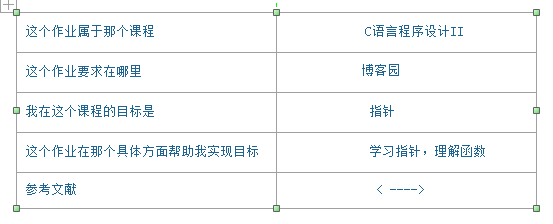基础题一
1.求两数平方根之和

double fun (double *a, double *b);

   #include<stdio.h>
#include <math.h>
double fun (double *a, double *b);
int main ( )
{ double a, b, y;
scanf ("%lf%lf", &a, &b );
y=fun(&a, &b); printf ("y=%.2f\n", y );
return 0;
}

/* 请在这里填写答案 */

   输入样例：
12 20
   输出样例：
y=7.94
2.试验代码：
#include<stdio.h>
#include <math.h>
double fun (double *a, double *b);
int main ( )
{ double a, b, y;
scanf ("%lf%lf", &a, &b );
y=fun(&a, &b); printf ("y=%.2f\n", y );
return 0;
}
double fun (double*a,double*b)
{
double y;
y=sqrt(*a)+sqrt(*b);
return y;
}

3.截图：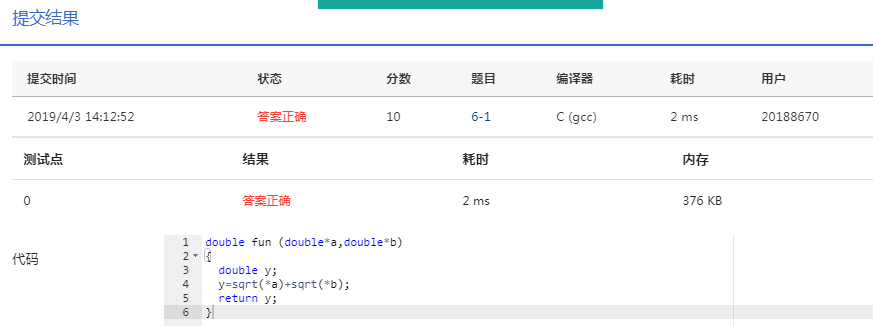4.本题问题不大
5.流程图：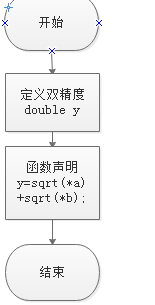### 基础题

利用指针返回多个函数值

    5
8 9 12 0 3
输出样例:

    max = 12
min = 0

2.代码：

#include<stdio.h>

void max_min(int n, int *a);

int main()
{
int n;
scanf("%d", &n);
int a[n];
for(int i = 0;i < n;i++)
{
scanf("%d", (a + i));
}

max_min(n, a);
return 0;
}

void max_min(int n, int *a)
{
int t;
for(int i = 0;i < n - 1;i++)
{
for(int j = 0;j < n - i - 1;j++)
{
if(*(a + j) < *(a + j + 1))
{
t = *(a + j + 1);
*(a + j + 1) = *(a + j);
*(a + j)= t;
}
}
}
printf("max = %d\n", *(a));
printf("min = %d\n", *(a + n - 1));

}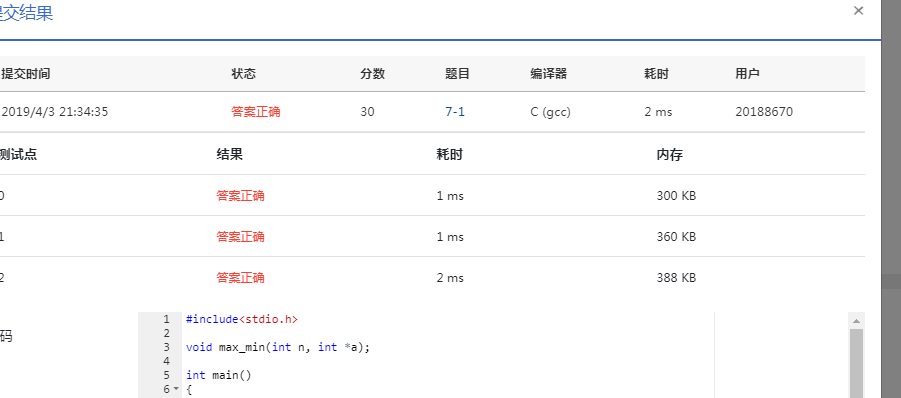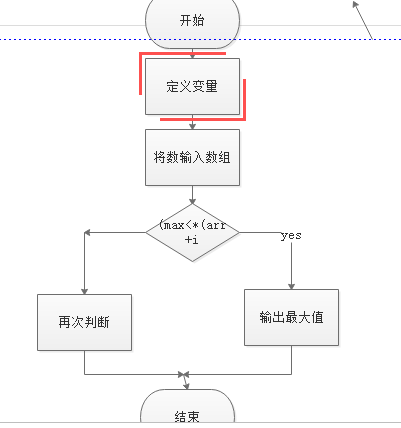预习题

void input(int *arr,int n);
void max_min(int *arr,int n);
void output(int *arr,int n);
三个函数中的 arr和n 都是用户传入的参数。n 是元素个数。

input函数的功能是输入 n个元素存到指针arr所指向的一维数组中。

max_min函数的功能是求指针arr所指向的一维数组中的最大值和最小值，其中最小的数与第一个数对换，将最大的数与最后一个数对换。

output函数的功能是在一行中输出数组元素，每个元素输出占3列。

#include<stdio.h>
void input(int *arr,int n);
void max_min(int *arr,int n);
void output(int *arr,int n);
int main()
{ int a;
input(a,10);
max_min(a,10);
output(a,10);
return 0;
}

/* 请在这里填写答案 */

    5 1 4 8 2 3 9 5 12 7
输出样例：
      1  5  4  8  2  3  9  5  7 12

 void input(int *arr,int n)
{
int i;
for(i=0;i<10;i++){
scanf("%d",arr+i);
}
}
void max_min(int *arr,int n)
{
int i,t=0;
int min=*arr,max=*arr,MAX,MIN;
for(i=1;i<10;i++)
if(min>*(arr+i)){
min=*(arr+i);
MIN=i;
}
t=*(arr+MIN);
*(arr+MIN)=*arr;
*arr=t;
for(i=1;i<10;i++)
if(max<*(arr+i)){
max=*(arr+i);
MAX=i;
}
t=*(arr+MAX);
*(arr+MAX)=*(arr+9);
*(arr+9)=t;
}
void output(int *arr,int n)
{
int i;
for(i=0;i<10;i++)
printf("%3d",*(arr+i));
}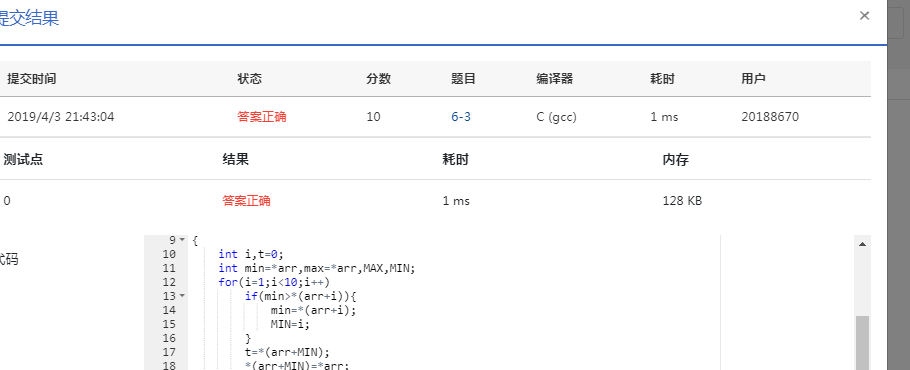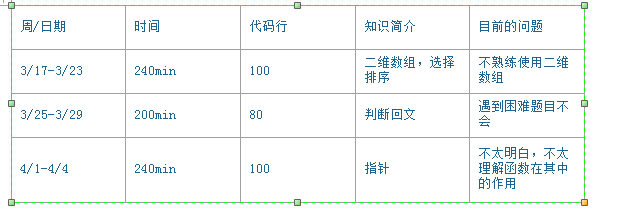### 为什么要使用指针？它有什么用？

1.指针就是指向一个特定内存地址的一个变量。C语言指针可以有效地表示复杂的数据结构、动态分配内存、高效地使用数组和字符串、使得调用函数时得到多个返回值等。指针的应用往往与数组联系在一起，是最频繁的，也是最基础的。在定义数组和函数时，系统都会为其自动分配一个指向其首地址的指针。对于一个数组，其名称就是一个指针变量。
2.指针能够有效的表示数据结构，能动态分配内存，能较方便的使用字符串，有效的使用数组。
3.指针直接与数据等的储存地址有关，是比较重要的。比如，值传递不如地址传递高效，因为值传递先从实参的地址中提出值，再赋值给形参带入函数计算；而指针则把形参的地址直接指向实参地址，使用时直接提出数据，使效率提高，特别在频繁赋值等情况下。